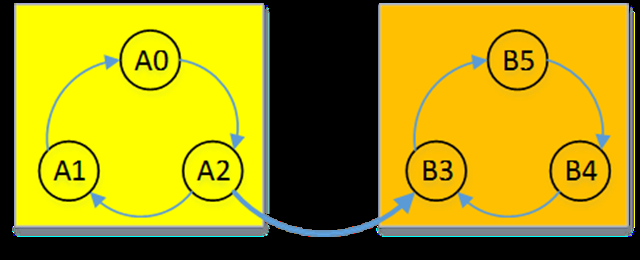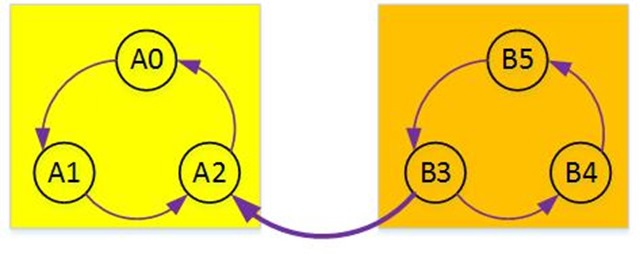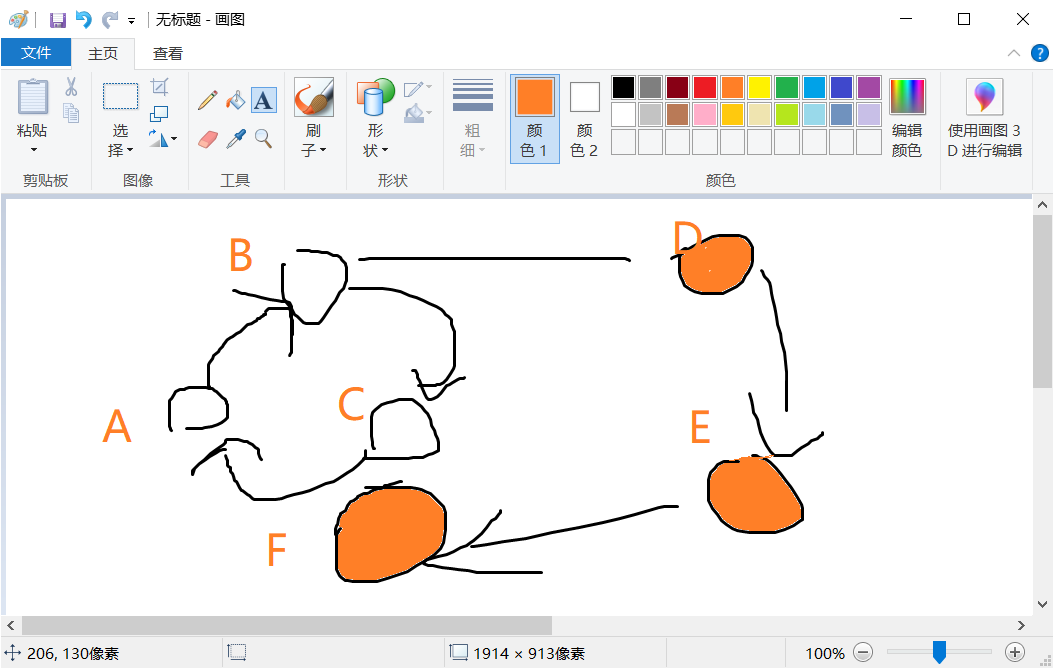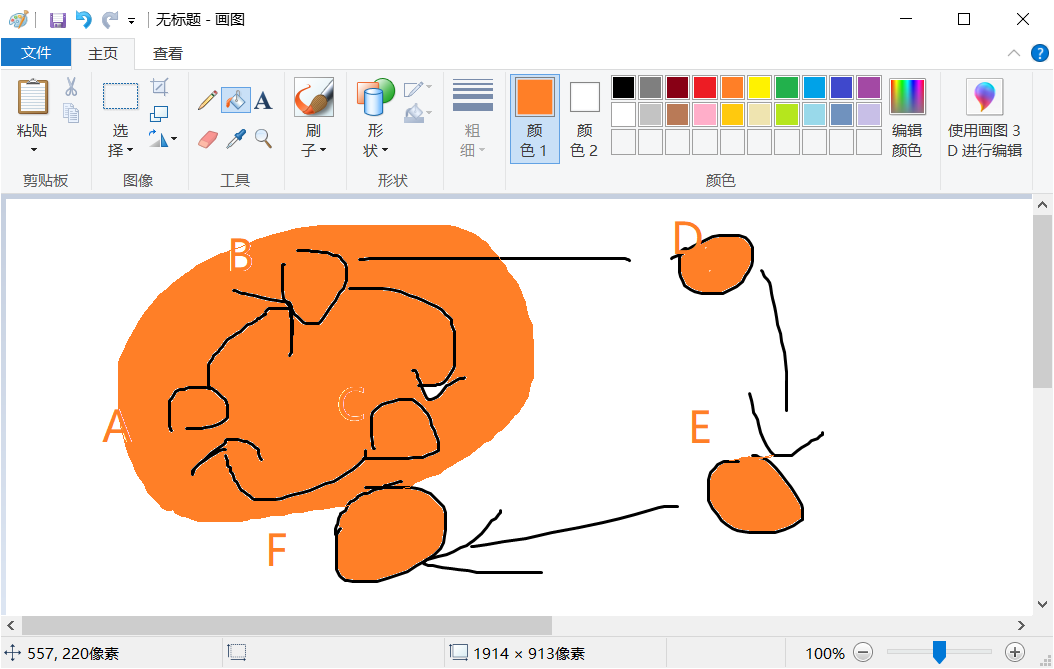•5星
6.43MB bala5569 2021-01-03 19:22:39
•5星
1.11MB weixin_41110581 2021-03-26 14:49:07
•5星
224KB weixin_42696333 2021-09-10 17:31:54
• 对于一个图，说他是连通的当且仅当从该图的任何一个顶点到该图的另一个顶点都走得通，强连通的意思是，对于一个有向图，任何一个顶点到另外一个顶点都能互通，强连通分量就是极大强连通子图，可以这么理解，对于该图...

https://byvoid.com/zhs/blog/scc-tarjan/，这里讲的tarjan已经很好了。

对于一个图，说他是连通的当且仅当从该图的任何一个顶点到该图的另一个顶点都走得通，强连通的意思是，对于一个有向图，任何一个顶点到另外一个顶点都能互通，强连通分量就是极大强连通子图，可以这么理解，对于该图的某个顶点，当他加入一个点能构成连通图时，就继续加点，直到加不了为止，此时构成的原图的一个子图就叫极大强连通子图，也叫强连通分量，一个图可以有多个强连通分量。求强连通分量的基本思想就是dfs，解法包括但不限于Tarjan算法、Kosaraju算法，其中kosaraju算法较为直观，易于理解，我上面挂的链接就是讲tarjan的。

下面给出一道例题以及AC代码：

http://poj.org/problem?id=1236

#pragma GCC optimize(2)

#include

#include

#include

#include

#include

#include

using namespace std;

typedef long long ll;

#define sc(z) scanf("%d", &(z))

#define _ff(i, a, b) for (int i = (a); i <= (b); ++i)

#define _f(i, a, b) for (int i = (a); i < (b); ++i)

#define _rr(i, a, b) for (int i = (a); i >= (b); --i)

#define _r(i, a, b) for (int i = (a); i > (b); --i)

#define pb push_back

const int inf = 0x3f3f3f3f;

const int maxn = 105;

vector G[maxn];

int dfn[maxn], low[maxn], dfncnt, s[maxn], instack[maxn], tp;

int ssc[maxn], sc, n, in[maxn], out[maxn];

void tarjan(int u) {

low[u] = dfn[u] = ++dfncnt, s[++tp] = u, instack[u] = 1;

_f(i, 0, G[u].size()) {

int v = G[u][i];

if (!dfn[v]) {

tarjan(v);

low[u] = min(low[u], low[v]);

} else if (instack[v]){

low[u] = min(low[u], dfn[v]);

}

}

if (dfn[u] == low[u]) {

++sc;

while (s[tp] != u) {

ssc[s[tp]] = sc;

instack[s[tp]] = 0;

--tp;

}

ssc[s[tp]] = sc;

instack[s[tp]] = 0;

--tp;

}

}

void solve() {

dfncnt = sc = tp = 0;

_ff(i, 1, n) if (!dfn[i]) tarjan(i);

}

signed main() {

int v; sc(n);

_ff(i, 1, n) while(sc(v) && v) G[i].pb(v);

solve();

_ff(i, 1, n)

_f(j, 0, G[i].size()) {

int k = G[i][j];

if (ssc[k] != ssc[i]) {

in[ssc[k]]++;

out[ssc[i]]++;

}

}

if (sc == 1) printf("1\n0");

else {

int x1 = 0, x2 = 0;

_ff(i, 1, sc) {

if (!in[i]) x1++;

if (!out[i]) x2++;

}

printf("%d\n%d", x1, max(x1, x2));

}

return 0;

}

展开全文weixin_39610415 2020-12-20 11:03:29
• 求有向图的强连通分量个数（kosaraju算法） kosaraju算法

有向图连通分量的求解思路 kosaraju算法 逛了很多博客，感觉都很难懂，终于找到一篇能看懂的，摘要记录一下 原博客https://www.cnblogs.com/nullzx/p/6437926.html 关于连通分量是什么自行百度，这里主要...

有向图的连通分量的求解思路

kosaraju算法

逛了很多博客，感觉都很难懂，终于找到一篇能看懂的，摘要记录一下

原博客https://www.cnblogs.com/nullzx/p/6437926.html

关于连通分量是什么自行百度，这里主要说明连通分量的求解方法基本思路：第一次DFS得出顶点的顺序，根据顶点顺序进行第二次DFS，也就是逆后序遍历（手动模拟一下堆栈就知道第二次DFS的过程就能得出答案）。

为什么要两次DFS?

如果从连通分量A中任意一个定点DFS，得不到正确结果。应该按照被指向的强连通分量的定点排在前面的顺序进行DFS。上图按照B3，B4，B5，A0，A1，A2的顺序DFS。实际中我们只要保证被指向的强连通分量的至少一个顶点排在指向这个连通分量的所有顶点前面即可，比如B3，A0，A1，A2，B4，B5；B3排在强连通分量A所有定点的前面。

如何得到满足要求的顶点顺序：对原图取反，从反向图的任意节点开始进行DFS的逆后序遍历

DFS的逆后序遍历指：如果当前顶点没被访问，先遍历完与当前顶点相连的且未被访问的所有其他顶点，然后将当前顶点加入栈，最后从栈顶到栈底的顺序是我们需要的顶点顺序。

其实它是利用了有向图的方向性：例如在上图中，强连通分量A和B不管正反图都能自己跑一圈，但是从A到B就只能从A2跑到B3，不可能从B3跑到A2，所以将图取反（注意图取反，不能从A2跑到B3，顶点顺序被记录，按顶点顺序一个个弹出遍历，之前遍历过的点就不用再遍历），做成反向图，再逆后序遍历，A0在栈顶，遍历一圈，不能从A2跑到B3，就得到一个连通分量（如下图）接下来原博主的代码看不懂，还是百度百科kosaraju算法里面的代码好

#include <iostream>
#include <cstring>
#include <cmath>
#include <cstdio>
#include <stack>
#include <algorithm>
using namespace std;
#define INF 999999999
#define MAXN 551
#define MOD 1000000009
int n;
int mp[MAXN][MAXN], nmp[MAXN][MAXN], vis[MAXN];
stack<int> s;
void dfs_1(int v)
{
//    cout << v <<endl;
vis[v] = 1;
for(int i = 1; i <= n; ++i)
if(!vis[i] && mp[v][i])
dfs_1(i);
s.push(v);
}
void dfs_2(int v)
{
vis[v] = 1;
for(int i = 1; i <= n; ++i)
if(!vis[i] && nmp[v][i])
dfs_2(i);
}
int kosaraju()
{
while(!s.empty())
s.pop();
memset(vis, 0, sizeof(vis));

for(int i = 1; i <= n; ++i)
if(!vis[i])
dfs_1(i);

int ans=0;
memset(vis, 0, sizeof(vis));

while(!s.empty())
{
int v = s.top();
s.pop();
if(!vis[v])
{
//            cout << v <<endl;
ans++;
dfs_2(v);
}
}
return ans;
}
int main()
{
int m, a, b;
//n个点，m条边
cin >> n >>m;
memset(mp, 0, sizeof(mp));
memset(nmp, 0, sizeof(nmp));
for(int i = 0; i < m; ++i)
{
cin >> a >>b;
mp[a][b] = 1;
nmp[b][a] = 1;
}
cout << kosaraju() <<endl;
return 0;
}
/*
5 5
1 2
2 1
2 3
3 4
4 1
样例输出：
2
*/

展开全文czsupercar 2019-04-02 16:14:29
• 关于有向图强连通分量 和 无向图双联通分量的理解 c++

有向图的强连通分量 1.强连通 代表的是 这个连通块中的每两个点互相都是有一条路径是可以走到的 2.分量 就是子图； 从这张图上可以看出 A B C这三个点都是互相可以走到的 所以他们就是一个联通块 D E F 三个点都是...

有向图的强连通分量
1.强连通 代表的是 这个连通块中的每两个点互相都是有一条路径是可以走到的
2.分量 就是子图；从这张图上可以看出 A B C这三个点都是互相可以走到的 所以他们就是一个联通块 D E F 三个点都是单向能走到 所以D E F 分别为三个联通分量
所以这个图中 一共有 四 个连通分量
然后又引入一个概念 缩点 将连通块中的点当成一个点
可以用来求 连通块问题我们知道概念了 该如何去求这个图中有几个连通分量呢
引用时间戳这个概念
A - > B -> D -> E -> F
A -> B -> C
所以ABCDEF的进入时间分别为1 2 6 3 4 5
我们走到 F的时候 发现没有路可以走了 所以没有形成 一个环所以 他自成一个 E D 分别自成一个 走到 C的时候发现可以回到 1 所以他是可以和 A成一个环的 所以 把C的时间戳修改成 A的时间戳 表明 他们是可以走一起的 同样返回的时候 把 B 的时间戳也修改成 与 C已经成功成环的时间戳 即可

#include<iostream>
#include<cstring>

using namespace std;

const int N = 1e4 + 10,M = 5e4 + 10;

to[++cnt] = b;
}

int n,m;
int toopc[N];
int dfn[N],low[N],sta[N],top,ttime,flag[N],scc_cnt,st_size[N],belong[N];
void dfs(int x){
dfn[x] = low[x] = ++ttime; //两个时间戳 一个记录进入时间 一个记录最后是否是一个时间戳的时间
sta[++top] = x,flag[x] = 1; //记录这个路径中的点
for(int i = head[x]; i != -1; i = last[i]){
int j = to[i];
if(!dfn[j]){
dfs(j);
low[x] = min(low[x],low[j]); //变成 下一个点已经成环的时间戳
}else if(dfn[j] && flag[j]){
low[x] = min(low[x],dfn[j]); //如果此次路劲已经走过了他 那么直接判断即可
}
}

if(low[x] == dfn[x]){ //如果时间戳相同的话 那么这个点就是这个环的起点位置
++scc_cnt;
int y;
int h = 0;
do{
++h;
y = sta[top--];
flag[y] = 0;
belong[y] = scc_cnt; //这个点属于这个环
}while(y != x);
st_size[scc_cnt] = h;
}
}

int main(){
cin >> n >> m;
for(int i = 1; i <= m; i++){
int x,y;
cin >> x >> y;
}

for(int i = 1; i <= n; i++){
if(!dfn[i]) dfs(i);
}

for(int i = 1; i <= n; i++){
for(int j = head[i]; j != -1; j = last[j]){
int k = to[j];
if(belong[i] != belong[k]){
toopc[belong[i]]++;
}
}
}

int sum = 0;int s = 0;
for(int i = 1; i <= scc_cnt; i++){
if(toopc[i] == 0){
sum += st_size[i];
++s;
}
if(s > 1){
sum = 0;
}
}

cout << sum << endl;
return 0;
}

双连通分量指的是 连通块中的每两个点都有 两条不相交的路径可以走到对方
而去掉图中的一条边 使连通块增加的这个边 叫做桥

去掉 全部与一个点的边 使连通块数量增加的点 叫割点红色的点就是割点

如何去求一个图中的桥呢由图推导
A->B->C->D->C 所以C和D是一个环 是一个联通块
但是C -> B 是B -> C 的反向边 所以 C->B不能使ABCD成为同一个双连通块
所以 这个时候 B->C就成为了一个桥同样B->C也是一个桥 所以当
这条边无法是他们成为同一个连通块的时候 就是一个桥；

#include<iostream>
#include<cstring>

using namespace std;

const int N = 1e4 + 10,M = 1e5 + 10;

int n,m;
to[cnt] = b;
}

int dfn[N],low[N],sta[N],is_bridge[N],belong[N],toop[N];
int times,top,scc_cnt;
void tarjan(int x,int lastt){
dfn[x] = low[x] = ++times;
sta[++top] = x;
for(int i = head[x]; i != -1; i = last[i]){
int j = to[i];
if(!dfn[j]){
tarjan(j,i);
low[x] = min(low[x],low[j]);
if(dfn[x] < low[j]){ // 如果low[j] > dfn[x] 说明 j 和 x点不是同一连通分量
is_bridge[i] = is_bridge[i ^ 1] = 1;
}
}else if(i != (lastt ^ 1)){ //由于是无向图所以 i + 1 是 i 的反向边 如果 i % 2 == 0
low[x] = min(low[x],dfn[j]);  //如果不判断反向边的话 dfn[x] == low[j]
}
}
if(dfn[x] == low[x]){
++scc_cnt;
int y;
do{
y = sta[top--];
belong[y] = scc_cnt;
}while(y != x);
}
}

int main(){
cin >> n >> m;
for(int i = 1; i <= m; i++){
int x,y;
cin >> x >> y;
}

tarjan(1,-1);

for(int i = 0; i < cnt; i++){
if(is_bridge[i]){
toop[belong[to[i]]]++;
}
}

int ans = 0;
for(int i = 1; i <= scc_cnt; i++){
if(toop[i] == 1){
ans++;
}
}

cout << (ans + 1) / 2 << endl;

return 0;
}

割点怎么求；
我们照样可以用图来推导C ->B -> A
我们发现 A自成了一个连通分量 所以A只能 通过 B才能返回到原来的祖先
所以B就是一个割点
同样如果A B 成环的话 A 还是得需要从B才能回到C 所以B还是割点
所以当dfn[i] <= low[j]的时候 那么B就是一个割点

#include<iostream>
#include<cstring>
#include<vector>

using namespace std;

const int N = 1010;
int n,maxn;

int head[N],to[N * 2],last[N * 2],cnt;
to[++cnt] = b;
}

vector<int>dcc[N];
int dfn[N],low[N],sta[N],cut[N];
int times,top,root,dcc_cnt;
void tarjan(int x){
dfn[x] = low[x] = ++times;
sta[++top] = x;
int ans = 0;
if(x == root && head[x] == -1){
++dcc_cnt;
dcc[dcc_cnt].push_back(x);
return;
}

for(int i = head[x]; i != -1; i = last[i]){
int j = to[i];
if(!dfn[j]){
tarjan(j);
low[x] = min(low[x],low[j]);
if(dfn[x] <= low[j]){
ans++;
if(x != root || ans > 1) cut[x] = 1; //如果他是根节点的话那么他就没有祖先所以 只有他有两个儿子节点才能变成割点
++dcc_cnt;
int y;
do{
y = sta[top--];
dcc[dcc_cnt].push_back(y);//将割点影响到的点记录下来
}while(y != j); //如果y != x的话 那么割点x就没了，那么后续如果还有连通分量的话 就不成立了
dcc[dcc_cnt].push_back(x);
}
}else low[x] = min(low[x],dfn[j]);
}
}

int main(){
int casse = 0;
while(cin >> n && n){
memset(dfn,0,sizeof dfn);
memset(cut,0,sizeof cut);
maxn = 0,cnt = 0;
dcc_cnt = 0,times = 0,top = 0;
for(int i = 1; i <= n; i++){
int x,y;
cin >> x >> y;
maxn = max(maxn,x);
maxn = max(maxn,y);
}

for(root = 1; root <= maxn; root++){
if(!dfn[root]){
tarjan(root);
}
}

int sum = 0;
for(int i = 1; i <= maxn; i++){
if(cut[i] == 1) sum++;
}

cout << sum << endl;
}
return 0;
}

展开全文qqqingyi 2020-12-25 18:15:19
• 121KB weixin_42520293 2018-06-22 15:01:33
• 有向图的强连通性 & Kosaraju算法（求有向图的强连通分量） 算法 数据结构

浅谈无向图的连通连通图是无向图的一个概念： 在无向图中，若从顶点 v1v_1v1​ 到顶点 v2v_2v2​ 有路径，则称顶点 v1v_1v1​ 与 v2v_2v2​ 是连通的； 如果图中任意一对顶点都是连通的，则称此无向图是...在有向图

浅谈无向图的连通性

连通图是无向图的一个概念

• 在无向图中，若从顶点 v 1 v_1 到顶点 v 2 v_2 有路径，则称顶点 v 1 v_1 v 2 v_2 是连通的；
• 如果图中任意一对顶点都是连通的，则称此无向图是连通图

对于一个非连通图，可以通过深度优先搜索或广度优先搜索来获取它的连通分量：从一个未访问过的节点开始进行深度优先或者广度优先搜索，其能到达的所有顶点及其相关的边构成的子图就是一个连通分量；直到访问完全部的节点，便可以获取该图的所有连通分量。

有向图强连通性的概念

在有向图 G = ( V , E ) G=(V,E) 中，若对于每一对顶点 v 1 v_1 v 2 v_2 ，都存在一条从 v 1 v_1 v 2 v_2 和从 v 2 v_2 v 1 v_1 的路径，则称此图是强连通图

相应地有 强连通分量（极大强连通子图） 的概念：设 E i ( 1 ≤ i ≤ r ) E_i(1\leq i \leq r) 是头、尾均在 V i V_i 中的边集，则子图 G i ( V i , E i ) G_i(V_i,E_i) 称为 G G 的一个强连通分量，简称强分量、强支

强连通图只有一个强连通分量，即其自身；非强连通的有向图有多个强连通分量。如上图可以分为 5 个强连通分量。

设从 v v 可到达（以 v v 为起点的所有有向路径的终点）的顶点集合为 T 1 ( G ) T_1(G) ，而到达 v v （以 v v 为终点的所有有向路径的起点）的顶点集合为 T 2 ( G ) T_2(G) ，则包含 v v 的强连通分量的顶点集合是： T 1 ( G ) ∩ T 2 ( G ) T_1(G)∩T_2(G)

强连通图的性质定理

定理：一个有向图是强连通的，当且仅当 G G 中有一个回路，它至少包含每个顶点一次。

• 充分性：如果 G G 中有一个回路，它至少包含每个顶点一次，则 G G 中任两个顶点都是互相可达的，故 G G 是强连通图。
• 必要性：如果有向图是强连通的，则任两个顶点都是相互可达，故必可做一回路经过图中所有顶点。若不然则必有一回路不包含某一顶点 v v ，并且 v v 与回路上的各顶点就不是相互可达，与强连通条件矛盾。

求有向图强连通分量—Korasaju算法

在计算科学中，Kosaraju的算法（又称为 Sharir Kosaraju算法）是一个线性时间(linear time)算法找到的有向图的强连通分量。它利用了一个事实，逆图（与各边方向相同的图形反转, transpose graph）有相同的强连通分量的原始图。

算法步骤：

• （1）深度优先遍历 G G （起点如何选择无所谓），并计算出 每 个 顶 点 u 的 结 束 时 间 d f n [ u ] \color{red}{每个顶点 u 的结束时间 dfn[u]} （即按递归写法出系统栈的顺序编号，完成时间这个编号和深度优先遍历序列是不同的）；
• （2）深度优先遍历 G G 的转置（反向）图 G T G^T ，选择遍历的起点时，按照顶点的结束时间从大到小进行。遍历的过程中，一边遍历，一边给顶点做分类标记，每找到一个新的起点，分类标记值就加 1。
• （3）第（2）步中产生的标记值相同的顶点构成深度优先森林中的一棵树，也即一个强连通分量。

这里提出了 每 个 顶 点 的 结 束 时 间 \color{red}{每个顶点的结束时间} 这个概念，这个编号和深度优先搜索的编号是不同的，在拓扑排序的 DFS实现（递归）中也用到了每个顶点的结束时间。在上图中，图 ( a ) (a) 表示有向图 G G ，要求其强连通分量：

• 假设从 ( a ) (a) 开始进行深度优先搜索（其实选哪个点都无所谓，最后得到的强连通分量是相通的），记录每个顶点的结束时间，结束时间如 ( b ) (b) 所示；
• 然后对 G G 进行反向得到 G T G^T ，按上一步得到的结束时间从大到小再对 G T G^T 进行深度优先搜索（普通的深度优先搜索）：
• 先从结束时间最晚的 a a 开始搜，可以搜索到 a , d , c a,d,c 三个节点，所以将 a , d , c a,d,c 最为一个强连通分量中的节点；
• 目前未访问的且结束时间最晚的节点是 b b ，所以再从 b b 开始对 G T G^T 进行深度优先搜索，可以搜到 b , e , f b,e,f ，所以将 b , e , f b,e,f 作为一个强连通分量中的节点；
• 至此，图 G T G^T 中没有未访问的节点，算法结束，共两个强连通分量，如 ( d ) (d) 所示。

伪代码：

/* 按弧的正向搜索，起点如何选择无所谓 */
int in_order[MAX_VEX] ;
void DFS(OLGraph *G , int v)  	 // 求每个节点的结束时间
{
ArcNode *p;
Count = 0;
visited[v] = TRUE;
in_order[count++]=v; 		 // 出系统栈的顺序，不是真的深度优先搜索序列
}

/* 对图G按弧的逆向进行搜索 */
void Rev_DFS(OLGraph *G , int v)
{
ArcNode *p;
visited[v] = TRUE;
printf(“%d”, v); /* 输出顶点 */
if(!visited[p->tailvex])
Rev_DFS(G, p->tailvex);
}

void Strongly_Connected_Component(OLGraph *G)
{
int k = 1, v, j;

/* 对图G正向遍历 */
for (v=0; v<G->vexnum; v++)
visited[v] = FALSE;
for (v=0; v<G->vexnum; v++)
if(!visited[v])
DFS(G, v);

/* 对图G逆向遍历 */
for (v=0; v<G->vexnum; v++)
visited[v] = FALSE;
for (j=G->vexnum-1; j>=0; j--) {
v = in_order[j];
if (!visited[v]) {
// 从这个点开始的深度优先搜索能搜到的节点都属于一个连通分量
printf(“\n第%d个连通分量顶点: ”, k++);
Rev_DFS(G, v)；
}
}
}

参考

展开全文qq_39583450 2020-11-08 10:34:06
• 简单算法-有向图的强连通分量 算法

weixin_57544905 2021-09-13 10:03:01
• 基于非递归算法的无向图连通分量的识别 无向图

23KB nanlouzc 2017-04-18 16:02:36
•5星
40KB feijiaogu7393 2021-07-05 15:29:43
• qq_33726635 2020-05-09 17:05:07
• 有向图强连通分量之Tarjan算法 强连通分量

Akatsuki__Itachi 2018-08-23 20:05:48
• pcc386115710 2020-01-21 01:42:41
• GodJing007 2019-07-27 15:20:03
• qq_43345204 2019-08-15 14:41:36
• 151KB m0_52957036 2020-02-18 10:13:32
• riba2534 2018-01-15 11:15:06
• xiao2yang1998 2017-12-30 15:37:46
• lanzijingshizi 2019-03-25 22:35:11
• 图的连通分量个数 数据结构 算法 链表 有向图 c语言

qq_43753724 2020-07-28 00:28:37
• 【alg4-有向图】Kosaraju算法（计算强连通分量） 算法

qq_41242680 2020-12-17 16:46:22
• 有向图的强连通分量 算法 深度学习 自动驾驶

SteveMC 2021-09-20 20:47:41
• 连通图 连通分量 并查集 数据结构

u013288190 2021-02-12 18:23:22
• jason_jiajin 2021-07-15 20:53:43
• Java实现有向图强连通分量的Tarjan算法 Java 有向图

a1439775520 2019-07-26 18:01:13
• weixin_44307065 2019-09-04 20:37:04
• C++学习笔记：有向图的强连通分量 强连通分量 强连通图 Kosaraju

qq_44013342 2019-05-31 13:55:33
• judyge 2015-04-12 18:53:34
• zhangxiaojiakele 2020-03-19 10:44:50
• u013288190 2021-03-08 08:58:01
• 图论算法——无向图的连通分量 无向图的连通分量 连通分量

yjw123456 2019-05-22 19:19:37...